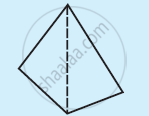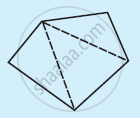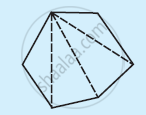Share

# Examine the Table. (Each Figure is Divided into Triangles and the Sum of the Angles Deduced from That.) What Can You Say About the Angle Sum of a Convex Polygon with Number of Sides? - CBSE Class 8 - Mathematics

ConceptPolygons Convex and Concave Polygons

#### Question

Examine the table. (Each figure is divided into triangles and the sum of the angles deduced from that.)

 FigureSide 3 4 5 6 Angle sum 180° 2 × 180° = (4 − 2) × 180° 3 × 180° = (5 − 2) × 180° 4 × 180° = (6 − 2) × 180°

What can you say about the angle sum of a convex polygon with number of sides?

a) 7

b) 8

c) 10

d) n

#### Solution

From the table, it can be observed that the angle sum of a convex polygon of nsides is (n −2) × 180º. Hence, the angle sum of the convex polygons having number of sides as above will be as follows.

(a) (7 − 2) × 180º = 900°

(b) (8 − 2) × 180º = 1080°

(c) (10 − 2) × 180º = 1440°

(d) (n − 2) × 180°

Is there an error in this question or solution?

#### APPEARS IN

NCERT Solution for Mathematics Textbook for Class 8 (2018 to Current)